# Intermediate Geometry : How to find the length of the side of an acute / obtuse triangle

## Example Questions

← Previous 1

### Example Question #1 : How To Find The Length Of The Side Of An Acute / Obtuse Triangle

In ΔABC: a = 10, c = 15, and B = 50°.

Find b (to the nearest tenth).

9.7

11.9

14.3

11.5

12.1

11.5

Explanation:

This problem requires us to use either the Law of Sines or the Law of Cosines. To figure out which one we should use, let's write down all the information we have in this format:

A = ?          a = 10

B = 50°       b = ?

C = ?          c = 15

Now we can easily see that we do not have any complete pairs (such as A and a, or B and b) but we do have one term from each pair (a, B, and c). This tells us that we should use the Law of Cosines. (We use the Law of Sines when we have one complete pair, such as B and b).

Law of Cosines: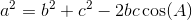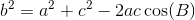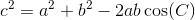Since we are solving for b, let's use the second version of the Law of Cosines.
This gives us: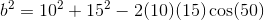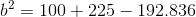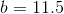### Example Question #2 : How To Find The Length Of The Side Of An Acute / Obtuse Triangle

In ΔABC, A = 35°, b = 8, c = 12.

Find a (to the nearest tenth).

5.3

8.3

7.1

9.0

6.8

7.1

Explanation:

This problem requires us to use either the Law of Sines or the Law of Cosines. To figure out which one we should use, let's write down all the information we have in this format:

A = 35°       a = ?

B = ?          b = 8

C = ?          c = 12

Now we can easily see that we do not have any complete pairs (such as A and a, or B and b) but we do have one term from each pair (A, b, and c). This tells us that we should use the Law of Cosines. (We use the Law of Sines when we have one complete pair, such as B and b).

Law of Cosines:Since we are solving for a, let's use the first version of the Law of Cosines.
This gives us: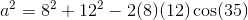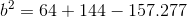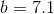### Example Question #3 : How To Find The Length Of The Side Of An Acute / Obtuse Triangle

In ΔABC, A = 25°, B = 50°, a = 17.

Find b (to the nearest tenth).

29.8

28.5

30.8

25.1

33.2

30.8

Explanation:

This problem requires us to use either the Law of Sines or the Law of Cosines. To figure out which one we should use, let's write down all the information we have in this format:

A = 25°       a = 17

B = 50°       b = ?

C = ?          c = ?

Now we can easily see that we have a complete pair, A and a. This tells us that we can use the Law of Sines. (We use the Law of Cosines when we do not have a complete pair).

Law of Sines: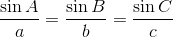To solve for b, we can use the first two terms which gives us: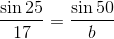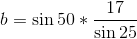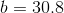### Example Question #4 : How To Find The Length Of The Side Of An Acute / Obtuse Triangle

Solve for. (Figure not drawn to scale)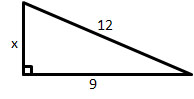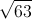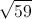Explanation:

Use the pythagorean theorem: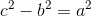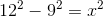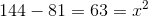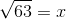### Example Question #5 : How To Find The Length Of The Side Of An Acute / Obtuse Triangle

A triangle has sides of lengths 18.4, 18.4, and 23.7. Is the triangle scalene or isosceles?

Scalene

Isosceles

Isosceles

Explanation:

The triangle has two sides of the same length, 18.4, so, by definition, it is isosceles.

### Example Question #6 : How To Find The Length Of The Side Of An Acute / Obtuse Triangle

A triangle has sides of lengths 12 meters, 1,200 centimeters, and 12 millimeters.  Is the triangle scalene, isosceles but not equilateral, or equilateral?

Isosceles, but not equilateral

Equilateral

Scalene

Isosceles, but not equilateral

Explanation:

Convert each of the three measures to the same unit; we will choose the smallest unit, millimeters.

One meter is equivalent to 1,000 millimeters, so 12 meters can be converted to millimeters by multiplying by 1,000: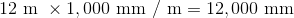One centimeter is equivalent to ten millimeters, so 1,200 cenitmeters can be converted to millimeters by multiplying by 10: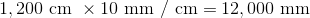These two sides have the same length. However, the third side, which has length 12 millimeters, is of different length. Since the triangle has exactly two congruent sides, it is by definition isosceles, but not equilateral.

### Example Question #1 : How To Find The Length Of The Side Of An Acute / Obtuse Triangle

A triangle has sides of lengths one and one half feet, twenty-four inches, and one yard. Is the triangle scalene, isosceles but not equilateral, or equilateral?

Scalene

Equilateral

Isosceles, but not equilateral

Scalene

Explanation:

Convert each of the three measures to the same unit; we will choose the smallest unit, inches.

One foot is equal to twelve inches, so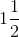feet can be converted to inches by multiplying by 12: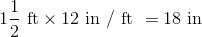One yard is equal to 36 inches.

The lengths of the sides in inches are 18, 24, and 36. Since no two sides have the same measure, the triangle is by definition scalene.

### Example Question #1 : How To Find The Length Of The Side Of An Acute / Obtuse Triangle

Two of the interior angles of a triangle have measure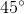and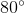. Is the triangle scalene or isosceles?

Isosceles

Scalene

Scalene

Explanation:

The measures of the interior angles of a triangle add up to. Ifis the measure of the third angle, then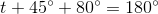Solve for: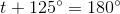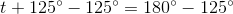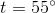By the Isosceles Triangle Theorem, if two sides of a triangle have the same length, their opposite angles have the same measure. Since no two angles have the same measure, no two sides have the same length. This makes the triangle scalene.

### Example Question #9 : How To Find The Length Of The Side Of An Acute / Obtuse Triangle

Two of the interior angles of a triangle have measure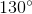and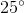. Is the triangle scalene or isosceles?

Scalene

Isosceles

Isosceles

Explanation:

The measures of the interior angles of a triangle add up to. Ifis the measure of the third angle, then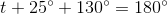Solve for: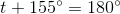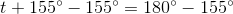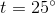The triangle has two congruent angles - each with measure. As a consequence, by the Converse of the Isosceles Triangle Theorem, the triangle has two congruent sides, making it, by definition, isosceles.

### Example Question #10 : How To Find The Length Of The Side Of An Acute / Obtuse Triangle

Two of the interior angles of a triangle have measure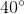. Is the triangle acute, right, or obtuse?

Obtuse

Right

Acute

Obtuse

Explanation:

The measures of the interior angles of a triangle add up to. Ifis the measure of the third angle, then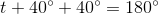Solve for: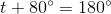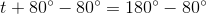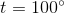The triangle therefore has an angle with measure greater than- an obtuse angle. The triangle is by definition an obtuse triangle,

← Previous 1